## LetsPlayMaths.Com

WELCOME TO THE WORLD OF MATHEMATICS

# Class 3 Money

Introduction to Money

Conversion of Rupees into Paise

Conversion of Paise into Rupees

Addition of Money Without Carry

Addition of Money With Carry

Subtraction of Money Without Borrow

Subtraction of Money With Borrow

Multiplication of Money by a Number

Division of Money by a Number

Money Test

Money Worksheet

Answer Sheet

## Introduction to Money

In India following coins are used.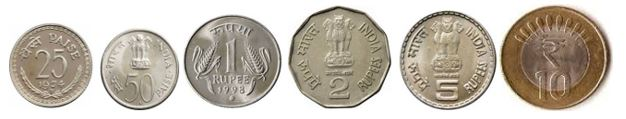Following currency notes are used in our country.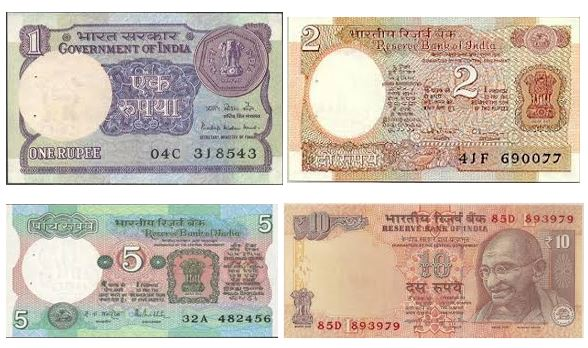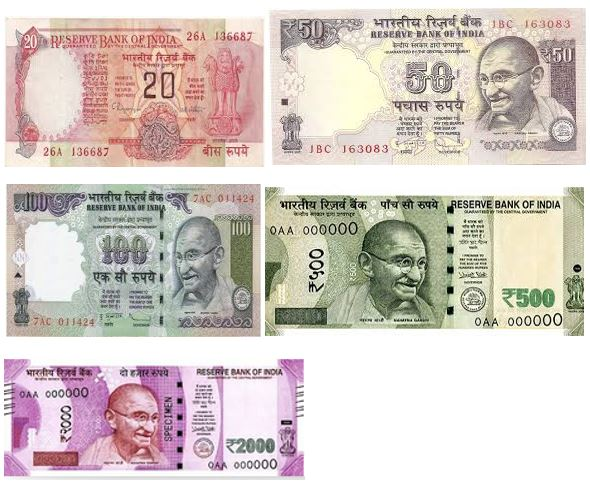Suppose you have 20 rupee note and 25 paisa coin. In words we express this amount as Rupees 20 and paisa 25.
In figures we write it as 20 Rupees 25 paise or ₹ 20.25.

₹ - International symbol for Rupees

35 rupees we write as Rs. 35 or ₹ 35.

When we write rupees and paise together, for example 25 rupees and 75 paise, we write ₹ 25.75. Rupees and paise are separated by a dot.

## Conversion of Rupees into Paise

As we know 1 Rupee = 100 Paise
To convert rupees into paise we have to multiply the number of rupees by 100

Example 1. Convert ₹ 45 into Paise

Solution. ₹ 45 = 45 X 100 = 4500 Paise

Example 2. Convert Rs 25.75 into paise

Solution. ₹ 25.75 = ₹ 25 + 75 P = 25X100P + 75P = 2500 + 75 = 2575 P

Another easy method, remove the point/dot and write ‘P’ at the end.
So, ₹ 25.75 = 2575P

## Conversion of Paise into Rupees

To convert paise into rupees and paise, the simple method is to put a dot (.) after two digits from right of the given number showing paise, and write Rs. On the left of the given number.

Example 1. Convert 425p to rupees and paise.

Solution. 425p = 400p + 25p

= ₹ 4 + 25p

= ₹ 4.25

Example 2. Convert 5665p to rupees and paise

Solution. 5665p = 5600p + 65p

= ₹ 56 + 65p

= ₹ 56.65

## Addition of Money Without Carry

Addition of money is like normal addition, here we must add the Paise first and then add the rupees. Have a look at the given examples.

Example 1. Add ₹ 35.20 and ₹ 5.25

Solution.Arrange the numbers in tabular format as shown below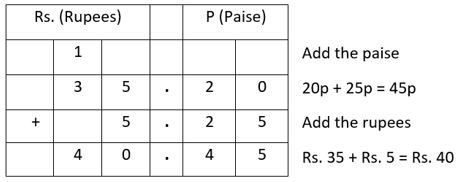So, the result is ₹ 40.45

Example 2. Add ₹ 432.22 to ₹ 147.65

Solution. Arrange the numbers in tabular format as shown below.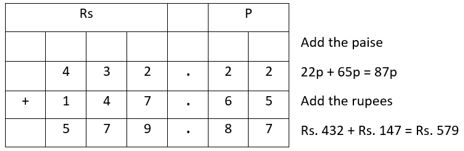So, the result is ₹ 579.87.

## Addition of Money With Carry

Addition of money with carry is like normal addition, here we have to add the Paise first, convert paise into rupees, and then add the rupees. In the Paise column, paise should always be written in two figures.
Have a look at the given examples.

Example 1. Add ₹ 86.57 and ₹ 52.66

Solution. Arrange the numbers in tabular format as shown below.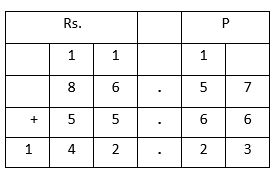Add the paise first

57p + 66p = 123p = 100p + 23p (100p = ₹ 1)

= ₹ 1 + 23p

23p to remain in paisa field.

Add the rupees now ₹ 86 + ₹ 55 + ₹ 1 = ₹ 142

So, the result is ₹ 142.23

Example 2. Add ₹ 132.45, ₹ 623.32 and ₹ 28.47

Solution. Arrange the numbers in tabular format as shown below.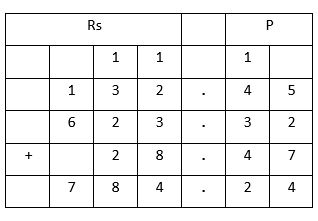So, the result is ₹ 784.24.

## Subtraction of Money Without Borrow

Subtraction of money is like normal subtraction, here we have to subtract the Paise first and then subtract the rupees. Have a look at the given examples.

Example 1. Subtract Rs. 234.23 from 987.56

Solution. Arrange the numbers in tabular format as shown below.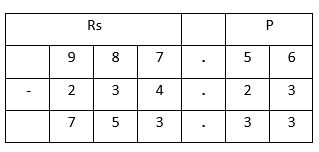Subtract the paise first

56p – 23p = 33p

Then subtract the rupees

₹ 987 – ₹ 234 = ₹ 753

So, the result is ₹ 753.33

## Subtraction of Money With Borrow

Let’s see an example to understand the process of subtraction with borrow.

Example 1. Subtract 242.35 from 353.21

Solution. Arrange the numbers in tabular format as shown below.
Method 1.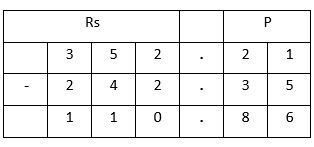Subtract the paise first

As 35p > 21p, borrow Rs 1 from 353.

1 rupee + 21p = 121 paise

121p – 35p = 86p

Write 86p below Paise column

Earlier ₹ 1 was borrowed from 353 then ₹ 352 remained. Subtract 242 from 352₹ 352 – ₹ 242 = ₹ 110

Write ₹ 110 below rupees column.

So, the answer is ₹ 110.86.

Method 2
Arrange the amount to be subtracted under the larger amount in a column such that dots fall in a column as shown below.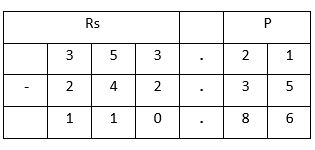Subtract the amounts like ordinary numbers and put a dot in the result dot’s column.

## Multiplication of Money by a Number

Multiplication of money is done in the same way as we multiply numbers, but we put a dot leaving two digits from the right. Let’s have a look at some examples.

Example 1. Multiply ₹ 24 by 5

Solution.So, the result is ₹ 120

Example 2. Multiply Rs. 9.52 by 5

Solution.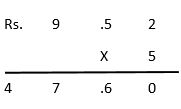So, the result is Rs. 47.60

Example 3. Multiply Rs. 15.12 by 12

Solution.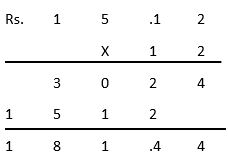So, the answer is Rs. 181.44.

## Division of Money by a Number

Division of money is done in the same way as we Divide numbers, but we put a dot leaving two digits from the right. Let’s have a look at some examples.

Example 1. Divide Rs. 35 by 7

Solution.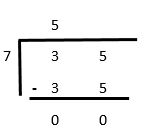So, the answer is 5.

Example 2. Divide 63.72 by 9

Solution.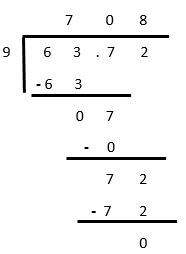So, the answer is Rs. 7.08

Money Test - 1

Money Test - 2

## Money Worksheet

Money Worksheet - 1

Money Worksheet - 2

Money Worksheet - 3

## Answer Sheet

Money-AnswerDownload the pdf

Copyright © 2021 LetsPlayMaths.com. All Rights Reserved.